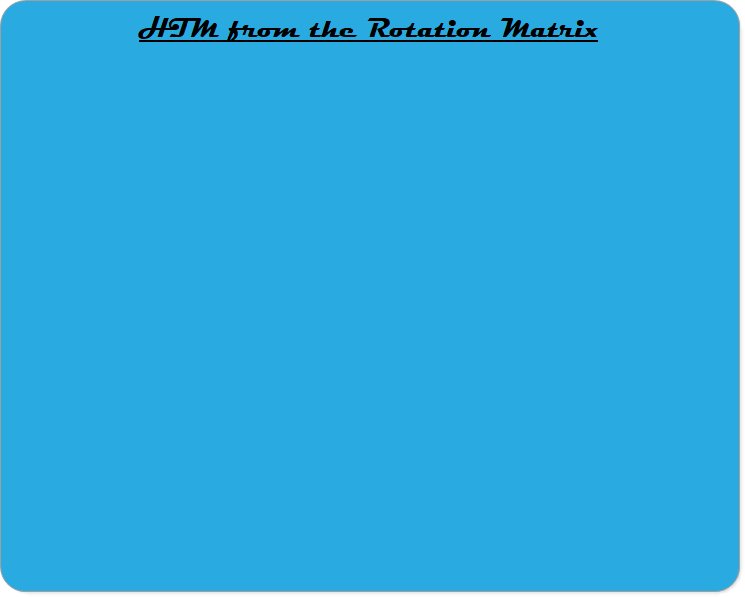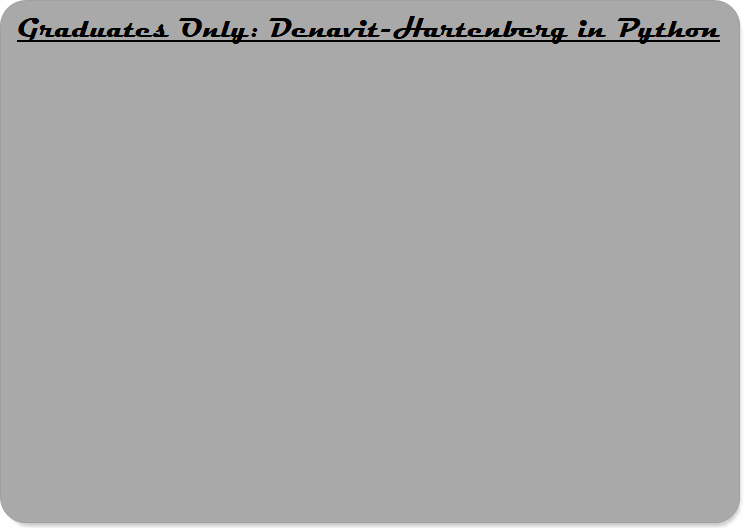Robotics 1
Homogeneous Transformation MatrixThis video shows how the rotation matrix and the displacement vector can be combined to form the Homogeneous Transformation Matrix. These matrices can be combined by multiplication the same way rotation matrices can, allowing us to find the position of the end-effector in the base frame.  In this video, we complete calculating the Homogeneous Transformation Matrix in our Python code and test the results with the manipulator we built on our board.
To complete this lab activity, make a video that includes the following in one video:

(2) Your 2-degree-of-freedom SCARA manipulator built on your board with the marker as its end-effector, moving to a position
(3) Your Python code correctly using the homogeneous transformation matrix to predict the position of the marker